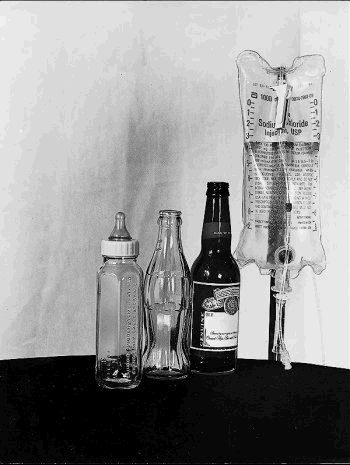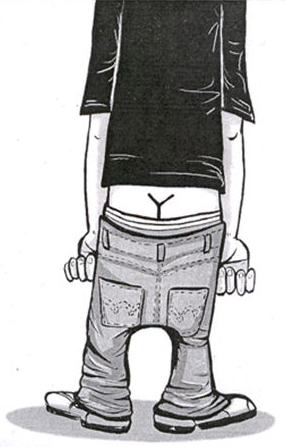Did  You  Know ?

• "Stewardesses" is the longest word that is typed with
only the left hand.

• Pearls melt in vinegar.

• An ostrich's eye is bigger than its brain.

• Snails can sleep for three years without eating.

• The highest point in Pennsylvania is lower than the
lowest point in Colorado.

• Ten percent of the Russian government's income comes from
the sale of vodka.

• the word FAMILY means?
FAMILY = Father  And  Mother  I
Love You.

• 13837 x Your age x 73 = ?

1 x 1 = 1
11 x 11 = 121
111 x 111 = 12321
1111 x 1111 = 1234321
11111 x 11111 = 123454321
111111 x 111111 = 12345654321
1111111 x 1111111 = 1234567654321
11111111 x 11111111 = 123456787654321
111111111 x 111111111 = 12345678987654321

1 x 8 + 1 = 9
12 x 8 + 2 = 98
123 x 8 + 3 = 987
1234 x 8 + 4 = 9876
12345 x 8 + 5 = 98765
123456 x 8 + 6 = 987654
1234567 x 8 + 7 = 9876543
12345678 x 8 + 8 = 98765432
123456789 x 8 + 9 = 987654321

1 x 9 + 2 = 11
12 x 9 + 3 = 111
123 x 9 + 4 = 1111
1234 x 9 + 5 = 11111
12345 x 9 + 6 = 111111
123456 x 9 + 7 = 1111111
1234567 x 9 + 8 = 11111111
12345678 x 9 + 9 = 111111111
123456789 x 9 +10= 1111111111

9 x 9 + 7 = 88
98 x 9 + 6 = 888
987 x 9 + 5 = 8888
9876 x 9 + 4 = 88888
98765 x 9 + 3 = 888888
987654 x 9 + 2 = 8888888
9876543 x 9 + 1 = 88888888
98765432 x 9 + 0 = 888888888

 9 X 1 = 09 9 X 2 = 18 9 X 3 = 27 9 X 4 = 36 9 X 5 = 45 9 X 6 = 54 9 X 7 = 63 9 X 8 = 72 9 X 9 = 81 9 X 10 = 90THE  FOUR  STAGES  OF  LIFE
The Silent generation, people born before 1946.

The Baby Boomers, people born between 1946 and 1959.

Generation X, people born between 1960 and 1979.

Generation Y, people born between 1980 and 1995.A B C D E F G H I J K L M N O P Q R S T U V W X Y Z 1 2 3 4 5 6 7 8 9 10 11 12 13 14 15 16 17 18 19 20 21 22 23 24 25 26

H
+A+R+D+W+O+R+K

8+1+18+4+23+15+18+11 = 98%

K+N+O+W+L+E+D+G+E

11+14+15+23+12+5+4+7+5 = 96%

A+T+T+I+T+U+D+E

1+20+20+9+20+21+4+5 = 100%

L+O+V+E+O+F+G+O+D

12+15+22+5+15+6+7+15+4 = 101%